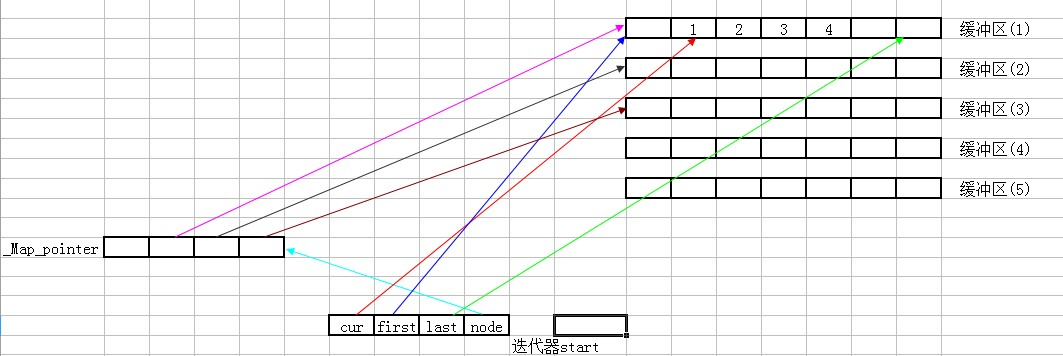# deque分析

May 18, 2013

#### 前言

deque元素的存储位置 首先可以从deque的迭代器代码入手。参见stl_deque.h文件，发现deque的迭代器与vector迭代器不同，它自己实现了一个`_Deque_iterator`迭代器，该迭代器中有几个比较重要的成员变量：

``````typedef _Deque_iterator _Self;
_Tp* _M_cur;  //当前元素
_Tp* _M_first;  //缓冲区头
_Tp* _M_last; //缓冲区尾
_Map_pointer _M_node;    //map节点
``````

iterator中有四个很重要的成员变量，`_M_cur`指向当前的元素，`_M_first`指向缓冲区的头部，`_M_last`指向缓冲区的尾部，`_M_node`是一个没有见过的变量（表面上看起来像是一个节点，但是一个什么节点呢？），实际上 `_M_node` 是一个typedef出来的：

``````typedef _Tp** _Map_pointer
````````````_Deque_base(const allocator_type& __a, size_t __num_elements)
: _Base(__a), _M_start(), _M_finish()
{
_M_initialize_map(__num_elements);
}
``````

``````template <class _Tp, class _Alloc>
void _Deque_base<_Tp,_Alloc>::_M_initialize_map(size_t __num_elements)
{
size_t __num_nodes = __num_elements / __deque_buf_size(sizeof(_Tp)) + 1;
_M_map_size = max((size_t) _S_initial_map_size, __num_nodes + 2);
_M_map = _M_allocate_map(_M_map_size);
_Tp** __nstart = _M_map + (_M_map_size - __num_nodes) / 2;
_Tp** __nfinish = __nstart + __num_nodes;
__STL_TRY
{
_M_create_nodes(__nstart, __nfinish);
}
__STL_UNWIND((_M_deallocate_map(_M_map, _M_map_size), _M_map = 0, _M_map_size = 0));
_M_start._M_set_node(__nstart);
_M_finish._M_set_node(__nfinish - 1);
_M_start._M_cur = _M_start._M_first;
_M_finish._M_cur = _M_finish._M_first + __num_elements % __deque_buf_size(sizeof(_Tp));
}
``````

#### deque的运作

``````void push_back(const value_type& __t)
{
if (_M_finish._M_cur != _M_finish._M_last - 1)
{
construct(_M_finish._M_cur, __t);
++_M_finish._M_cur;
}
else
_M_push_back_aux(__t);
}
``````

``````template <class _Tp, class _Alloc>
void deque<_Tp,_Alloc>::_M_push_back_aux(const value_type& __t)
{
value_type __t_copy = __t;
_M_reserve_map_at_back();
*(_M_finish._M_node + 1) = _M_allocate_node();
__STL_TRY
{
construct(_M_finish._M_cur, __t_copy);
_M_finish._M_set_node(_M_finish._M_node + 1);
_M_finish._M_cur = _M_finish._M_first;
}
__STL_UNWIND(_M_deallocate_node(*(_M_finish._M_node + 1)));
}
``````

`_M_reserve_map_at_back()`函数先不管，往下看。`_M_allocate_node()`配置了一个缓冲段，然后构造要push进去的元素，接下来`_M_set_node`改变迭代器finish的指向，使之指向新allocate的缓冲段，然后将当前元素指针指向push进去的元素。push_back的操作就这么做完了，如果是`push_front()`向deque的前端push一个元素呢？

``````void push_front(const value_type& __t)
{
if (_M_start._M_cur != _M_start._M_first)
{
construct(_M_start._M_cur - 1, __t);
--_M_start._M_cur;
}
else
_M_push_front_aux(__t);
}
``````

``````template <class _Tp, class _Alloc>
void  deque<_Tp,_Alloc>::_M_push_front_aux(const value_type& __t)
{
value_type __t_copy = __t;
_M_reserve_map_at_front();
*(_M_start._M_node - 1) = _M_allocate_node();
__STL_TRY
{
_M_start._M_set_node(_M_start._M_node - 1);
_M_start._M_cur = _M_start._M_last - 1;
construct(_M_start._M_cur, __t_copy);
}
__STL_UNWIND((++_M_start, _M_deallocate_node(*(_M_start._M_node - 1))));
}
``````

`_M_reserve_map_at_front`先不管，同样`_M_allocate_node`开辟了一个缓冲段，然后让start迭代器指向新开辟的缓冲段的首地址，然后_M_cur 指针指向所要push进元素的位置，然后构造要push进去的位置（将元素push到`_M_cur` 所指向的位置），就这样push_front的工作完成，it’s ok!

``````void _M_reserve_map_at_back (size_type __nodes_to_add = 1)
{
if (__nodes_to_add + 1 > _M_map_size - (_M_finish._M_node - _M_map))
_M_reallocate_map(__nodes_to_add, false);
}
void _M_reserve_map_at_front (size_type __nodes_to_add = 1)
{
if (__nodes_to_add > size_type(_M_start._M_node - _M_map))
_M_reallocate_map(__nodes_to_add, true);
}

template <class _Tp, class _Alloc>
void deque<_Tp,_Alloc>::_M_reallocate_map(size_type __nodes_to_add,
bool __add_at_front)
{
size_type __old_num_nodes = _M_finish._M_node - _M_start._M_node + 1;
//__old_num_nodes map中已有的node，__nodes_to_add 需要add进去的node（即新开辟的缓冲段的个数）
size_type __new_num_nodes = __old_num_nodes + __nodes_to_add;
_Map_pointer __new_nstart;
if (_M_map_size > 2 * __new_num_nodes)
{
__new_nstart = _M_map + (_M_map_size - __new_num_nodes) / 2
+ (__add_at_front ? __nodes_to_add : 0);
if (__new_nstart < _M_start._M_node)
copy(_M_start._M_node, _M_finish._M_node + 1, __new_nstart);
else
copy_backward(_M_start._M_node, _M_finish._M_node + 1,
__new_nstart + __old_num_nodes);
}
else
{
size_type __new_map_size = _M_map_size + max(_M_map_size, __nodes_to_add) + 2;
_Map_pointer __new_map = _M_allocate_map(__new_map_size);
__new_nstart = __new_map + (__new_map_size - __new_num_nodes) / 2
+ (__add_at_front ? __nodes_to_add : 0);
copy(_M_start._M_node, _M_finish._M_node + 1, __new_nstart);
_M_deallocate_map(_M_map, _M_map_size);

_M_map = __new_map;
_M_map_size = __new_map_size;
}

_M_start._M_set_node(__new_nstart);
_M_finish._M_set_node(__new_nstart + __old_num_nodes - 1);
}
``````

#### deque元素的操作

``````void pop_back()
{
if (_M_finish._M_cur != _M_finish._M_first)
{
--_M_finish._M_cur;
destroy(_M_finish._M_cur);
}
else
_M_pop_back_aux();
}
template <class _Tp, class _Alloc>
void deque<_Tp,_Alloc>::_M_pop_back_aux()
{
_M_deallocate_node(_M_finish._M_first);
_M_finish._M_set_node(_M_finish._M_node - 1);
_M_finish._M_cur = _M_finish._M_last - 1;
destroy(_M_finish._M_cur);
}
void pop_front()
{
if (_M_start._M_cur != _M_start._M_last - 1)
{
destroy(_M_start._M_cur);
++_M_start._M_cur;
}
else
_M_pop_front_aux();
}
template <class _Tp, class _Alloc>
void deque<_Tp,_Alloc>::_M_pop_front_aux()
{
destroy(_M_start._M_cur);
_M_deallocate_node(_M_start._M_first);
_M_start._M_set_node(_M_start._M_node + 1);
_M_start._M_cur = _M_start._M_first;
}
``````

pop_back，pop_front内部代码比较简单，主要还是`_M_pop_back_aux``_M_pop_front_aux`这两个函数，先说`_M_pop_back_aux`函数，当所要pop的元素在某一个缓冲段首地址时，需要检查此时deque是否还有其他的缓冲段和元素所在缓冲段中是否有其他元素，如果有则free了元素之后需要将空的缓冲段也free掉（deque默认无值情况下只留下一个缓冲段），如果只有一个缓冲段就将这个元素free掉，然后更改start和finish迭代器状态（缓冲段不free）。同样，当pop的元素在finsih部分时，也需要做和上面一样的检查操作，这样做的保证了deque在最初的状态、无任何值得情况下都会只有一个缓冲段存在，节省空间，避免产生内存碎片。 clear函数比较简单，就是清除了整个deque中的元素留下一个缓冲段。（具体代码可以参见源代码）

erase函数是清除某一个元素，由于缓冲段内的元素是线性存储，因此在erase了某一个元素之后需要将元素移动，保证缓冲段内元素位置为空的地方要么在finish部分，要么在start部分。（具体代码可以参见源代码） 下面的一段傻逼代码主要就是deque中的常用操作。

``````void operatorDeque()
{
std::deque<int> de(10,0);
std::cout << "de内值初始化为0："<< endl;
for (int i = 0; i < de.size(); i++)
{
std::cout << de[i] << ",";
}
std::cout << endl;
for (int i = 0; i < de.size(); i++)
{
de[i] = i;
}
std::deque<int>::iterator iter;
iter = de.begin();
de.insert(iter,11);
iter = de.end();
de.insert(iter,22);
std::cout << "输出："<< endl;
for (int i = 0; i < de.size(); i++)
{
std::cout << de[i] << ",";
}
de.pop_back();
de.push_back(33);
de.push_front(44);
iter = find(de.begin(),de.end(),5);
de.erase(iter);
std::cout << "操作之后输出：" << endl;
for (int i = 0; i < de.size(); i++)
{
std::cout << de[i] << ",";
}
de.clear();
std::cout << "清除之后size:" << de.size() << endl;
}
``````